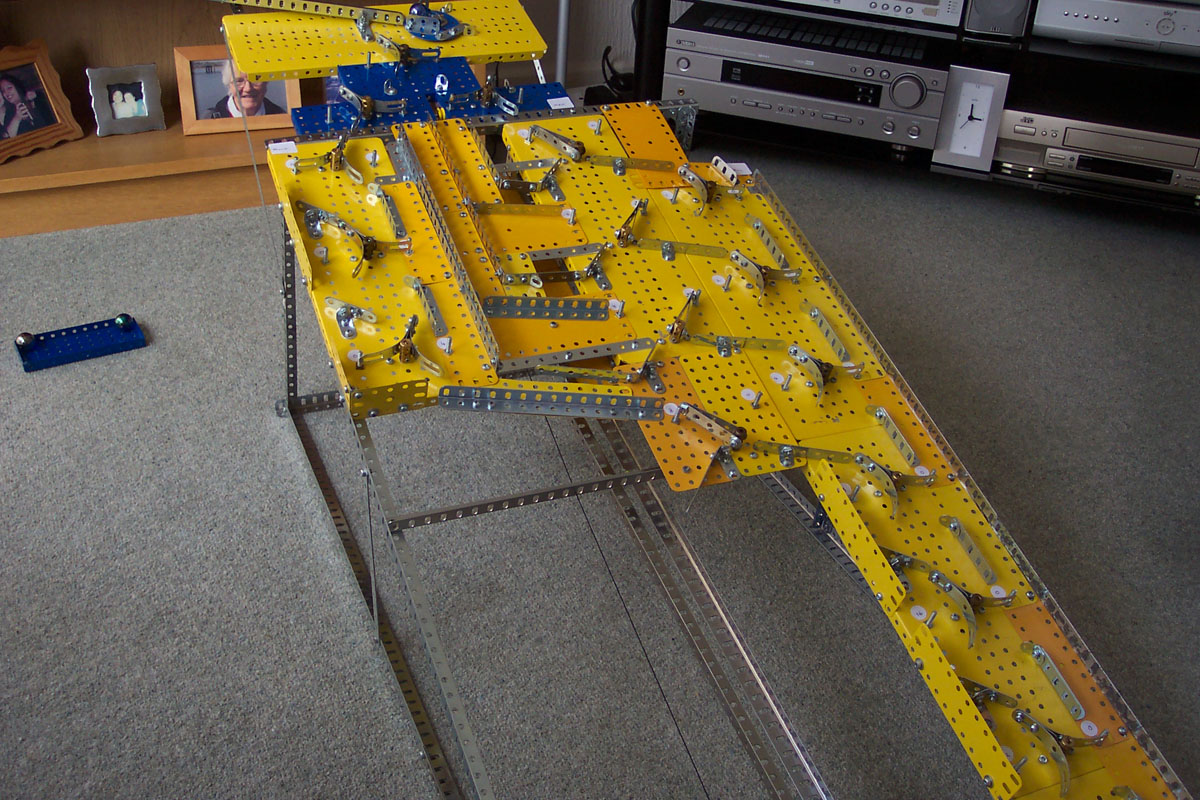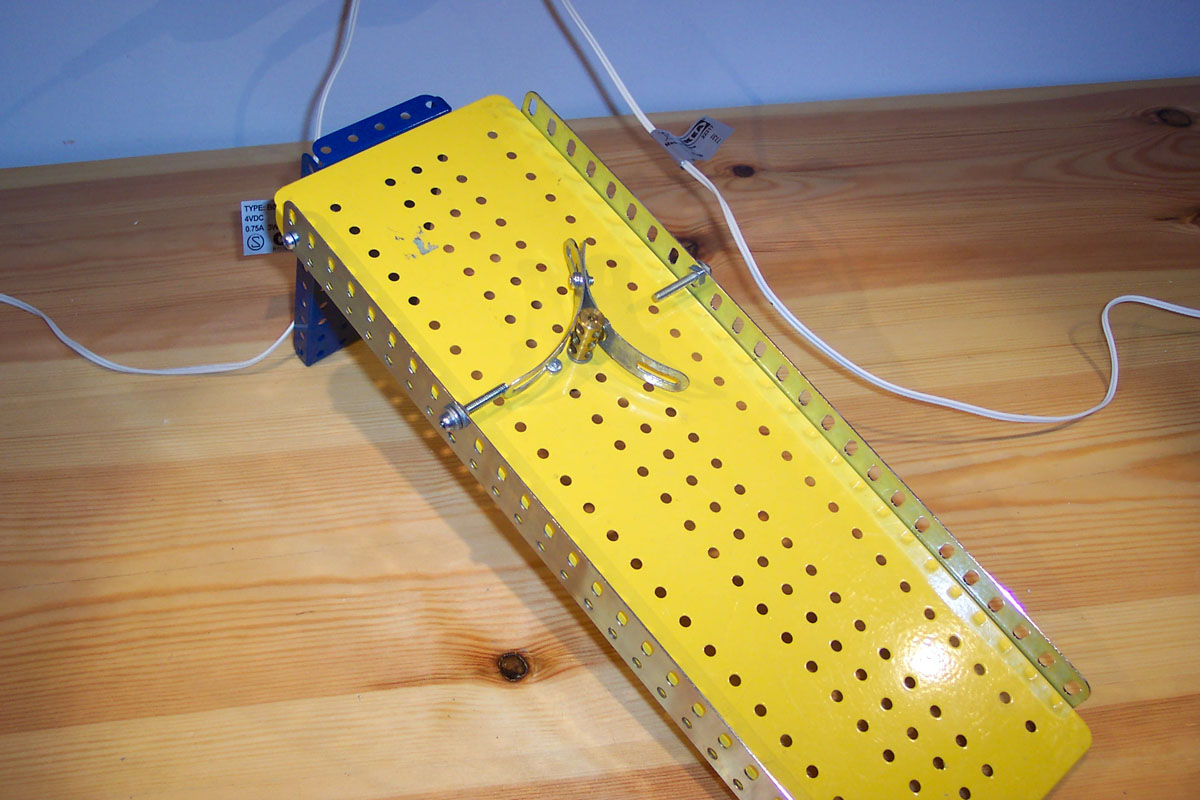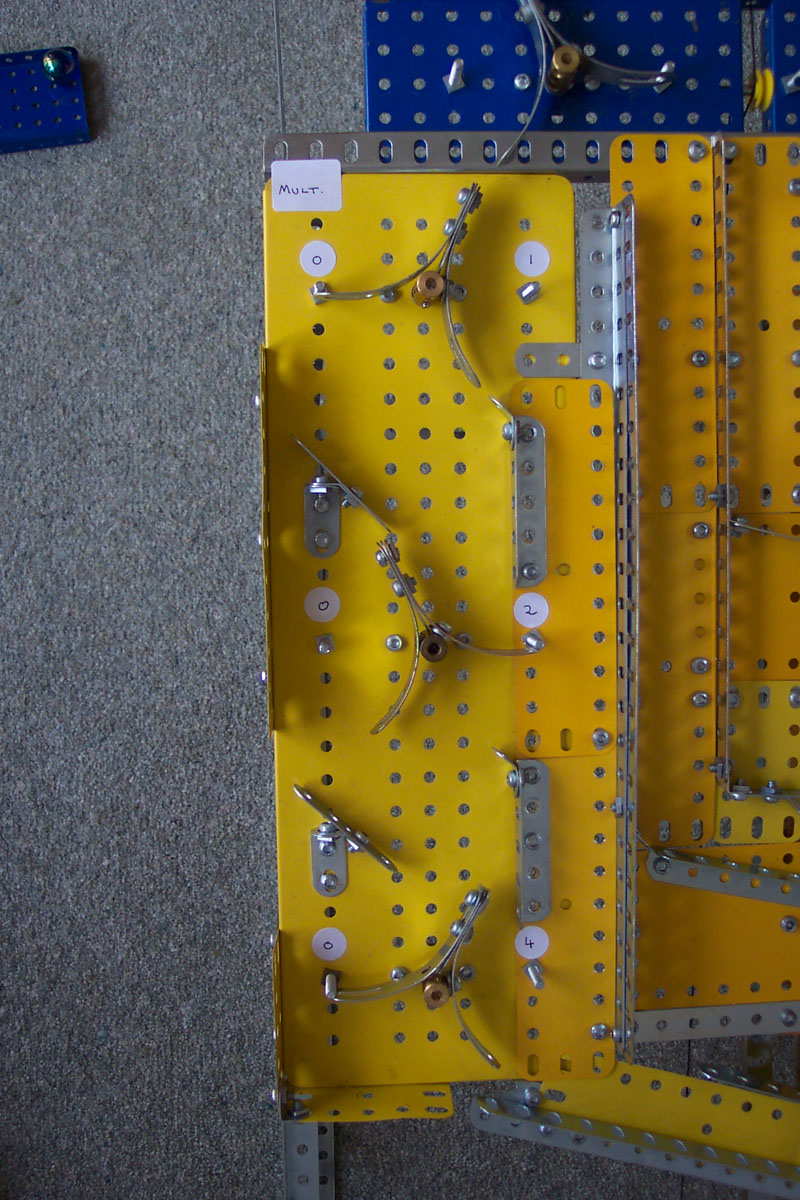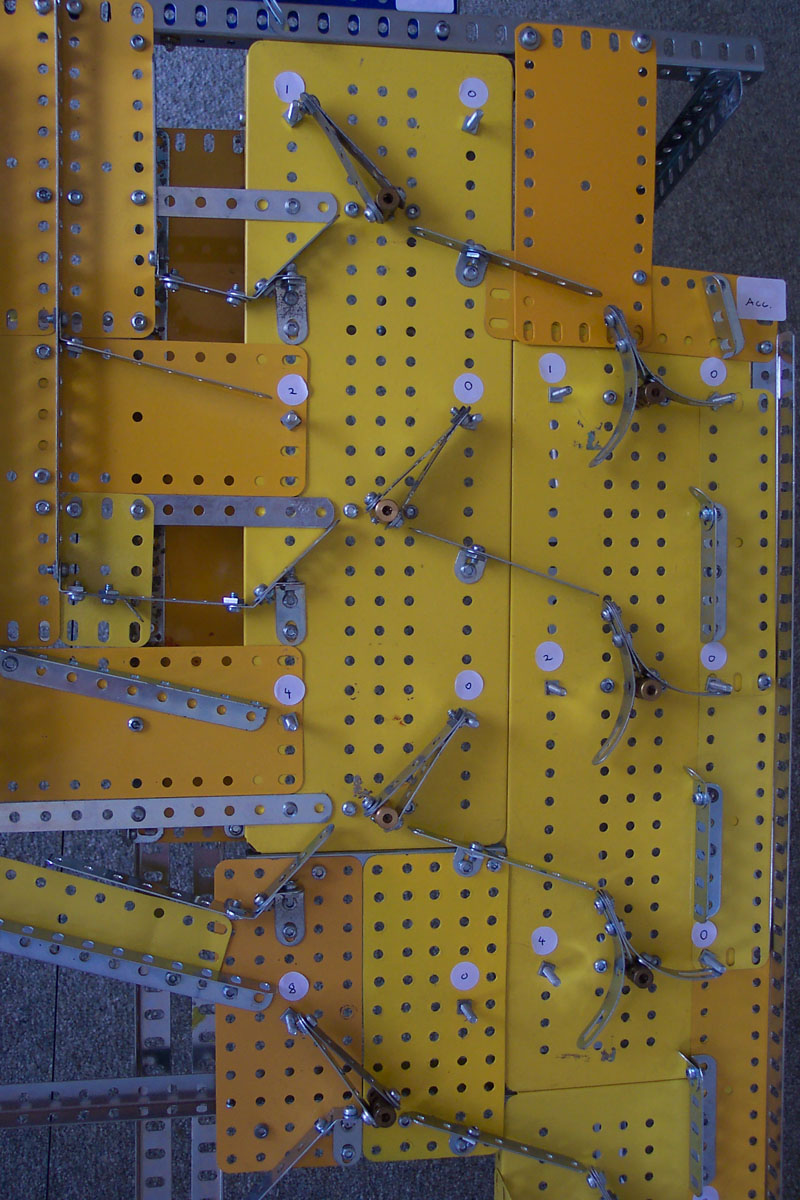Digicomp

This is a model of the Digicomp toy from the 1960s. It is a mechanical digital computer, which uses "flip flop" gates to represent binary numbers and perform calculations. A series of marbles run through the model changing the state of the flip flops.

There is a video of the model in operation further down this page.The computer is comprised of a series of flip flop gates - the picture below is a prototype. In the picture below, a marble will run down the left of the gate, but in doing so will flip it to the right. The next marble would then run down the right of the gate.The model has more limited functionality compared to the toy, as it can only multiply and add (not divide or subtract).

The multiplier register is on the left of the computer. In the picture below, it holds the number 2 (0 in the 1s place, 1 in the 2s place and 0 in the 4s place).The memory register is in the centre of the computer and the accumulator is on the right. In the picture below, the memory register holds the number 9 (1 in the 1s place, 0 in the 2s place, 0 in the 4s place and 1 in the 9s place).

For multiplication, the number in the multiplier register is multiplied by the number in the memory register.

The accumulator is set to 0 but will hold the result of the calculation when completed.At the top of the model is the distributor which is made up of three flip flop gates. On a complete cycle (where the distributor returns to its initial state), four marbles run through the distributor and run through each of the gates in the memory register once.

If the gate in the memory register is set to 0, the marble will run straight to the bottom of the computer. However, if the gate in the memory register is not set to 0, the marble runs into the accumulator.

At the start of each cycle of the distributor, the marble runs through the multiplier register, reducing its count by one each time. If this results in the multiplier register reaching zero, the calculation is complete and the marble comes to a rest. If the multiplier register is not zero, the marble runs into the multiplier register as normal.

Here is video of the model in operation, calculating 2 x 9 as set up in the pictures above:

A video overview of the model before doing the calculation shown in the video above: